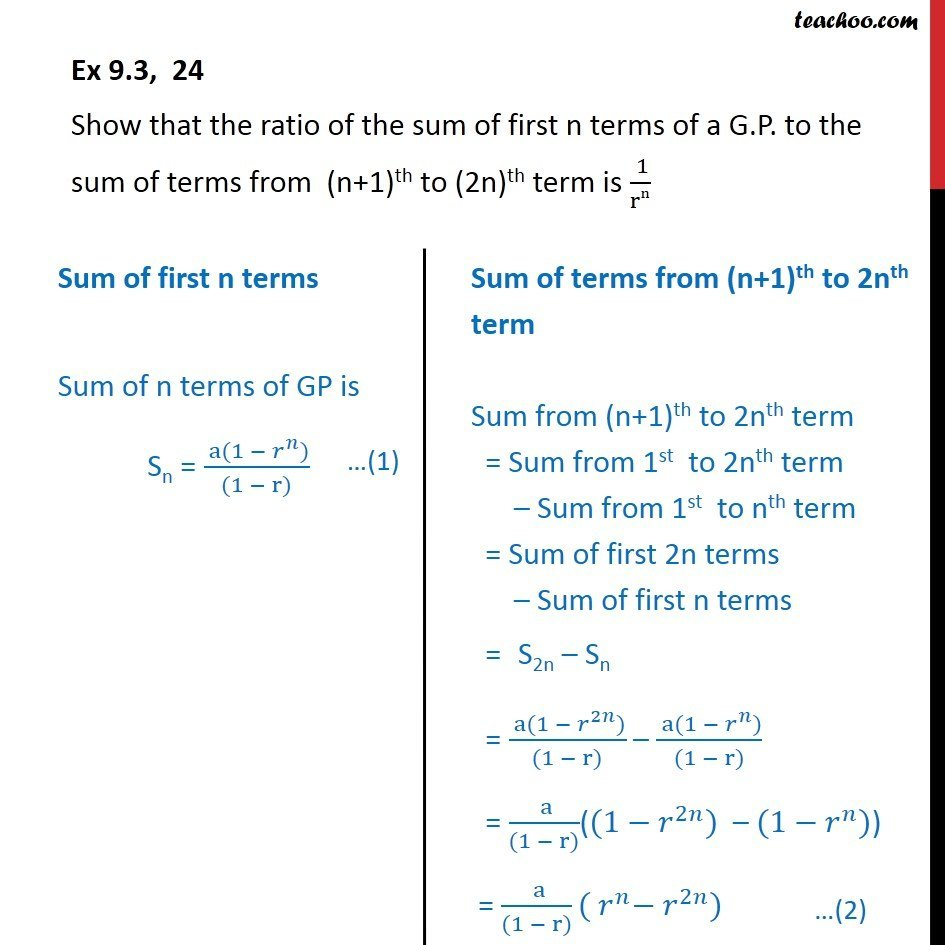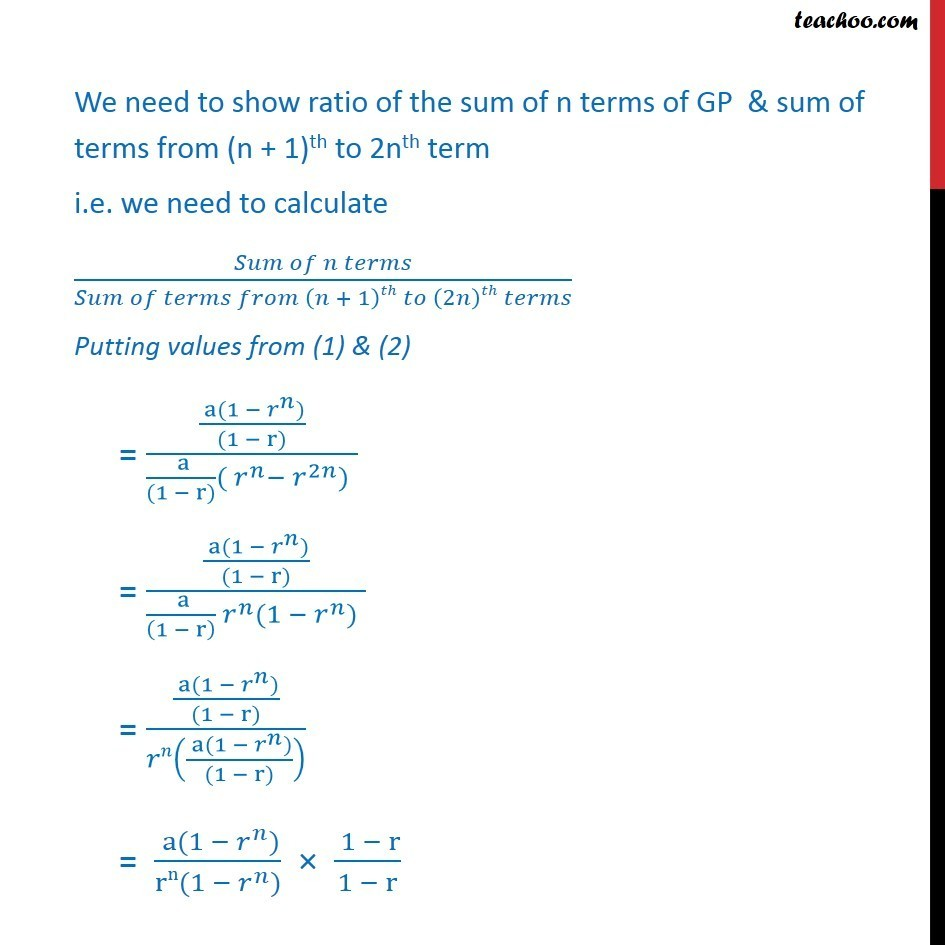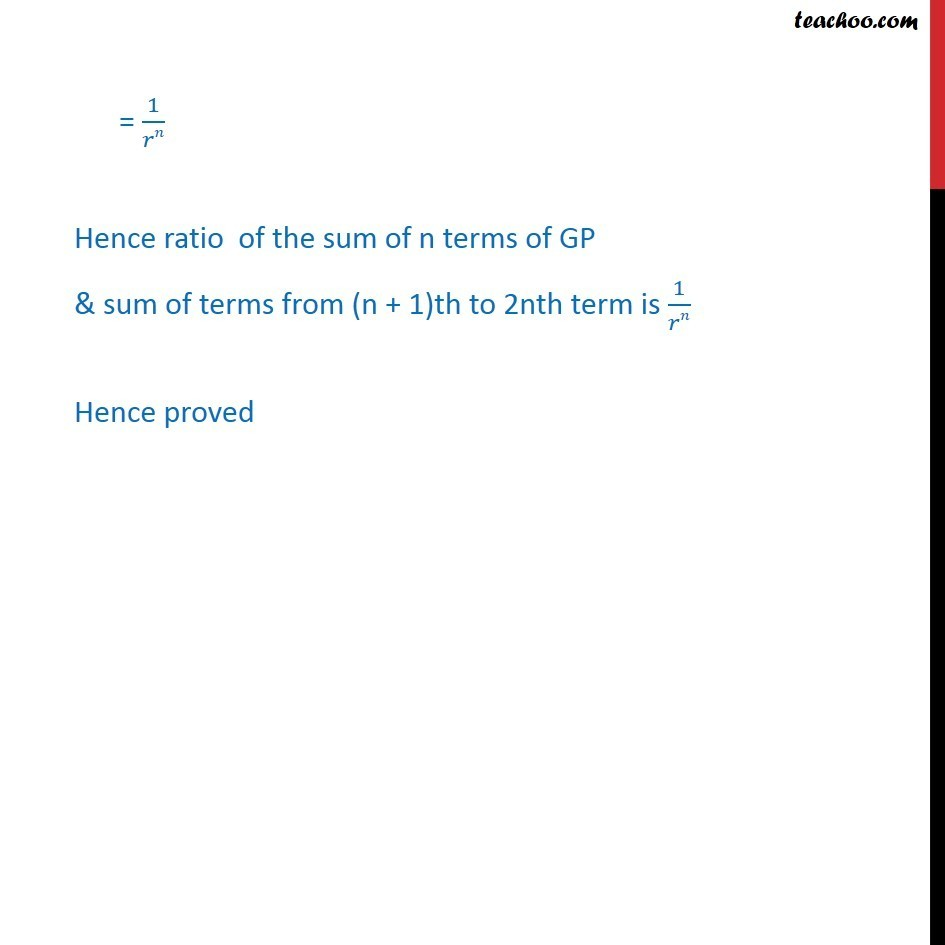Ex 8.2

Chapter 8 Class 11 Sequences and Series
Serial order wiseLearn in your speed, with individual attention - Teachoo Maths 1-on-1 Class

### Transcript

We need to show ratio of the sum of n terms of GP & sum of terms from (n + 1)th to 2nth term i.e. we need to calculate ( )/( ( + 1) (2 ) ) Putting values from (1) & (2) = (( a(1 ^ ))/((1 r)))/(( a)/((1 r))( ^ ^2 )" " ) = (( a(1 ^ ))/((1 r)))/(( a)/((1 r) ) ^ (1 ^ )" " ) = (( a(1 ^ ))/((1 r)))/ (( a(1 ^ ))/((1 r))) = ( a(1 ^ ))/(rn(1 ^ )) ( 1 r)/(1 r) Ex 8.2, 24 Show that the ratio of the sum of first n terms of a G.P. to the sum of terms from (n+1)th to (2n)th term is ( 1)/rn Ex 8.2, 24 Show that the ratio of the sum of first n terms of a G.P. to the sum of terms from (n+1)th to (2n)th term is ( 1)/rn We need to show ratio of the sum of n terms of GP & sum of terms from (n + 1)th to 2nth term i.e. we need to calculate ( )/( ( + 1) (2 ) ) Putting values from (1) & (2) = (( a(1 ^ ))/((1 r)))/(( a)/((1 r))( ^ ^2 )" " ) = (( a(1 ^ ))/((1 r)))/(( a)/((1 r) ) ^ (1 ^ )" " ) = (( a(1 ^ ))/((1 r)))/ (( a(1 ^ ))/((1 r))) = ( a(1 ^ ))/(rn(1 ^ )) ( 1 r)/(1 r) = 1/